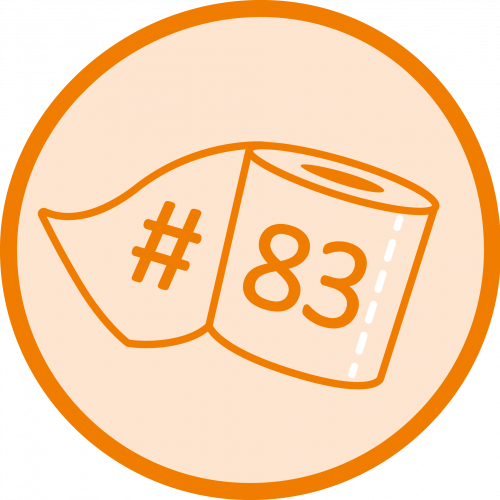Data visualization with D3.js

Problem

We want to display data files attractively in the browser. The diagram should be updateable, interactive and animatable

Solution

D3.js is a JavaScript library which allows generating dynamic, interactive diagrams for the browser. D3.js is not exactly a diagram library but rather a toolkit for generating various data visualizations.

How does D3.js work? For some time, browsers have been able to display SVG files with data models based on XML. D3.js takes advantage of this fact and generates or changes the DOM of the SVG file. Canvas and HTML are supported as well.

Example

SVG

Here is an example SVG for a small bar diagram:

<svg width="100" height="100" style="background:orange;">

<rect y="78" x="10" height="22" width="20"></rect>

<rect y="41" x="40" height="59" width="20"></rect>

<rect y="16" x="70" height="84" width="20"></rect>

</svg>

D3.js

JavaScript code for generating the diagram:

let height = 100, barWidth=20, padding=10;
let y = d3.scaleLinear() // 1) Value projection
.domain([1, 50])
.rangeRound([height, 0]);
d3.select('svg')
.attr('width', 100)
.attr('height', 100)
.attr('style', 'background:orange;')
.selectAll('rect') // 2) Selection
.data([12, 30, 42]) // 3) Data binding
.enter()
.append('rect') // 4) SVG DOM
.attr('y', (d) => {return y(d);})
.attr('x', (d,i) => {
})
.attr('height', (d) => {return height-y(d);})
.attr('width',barWidth);

1. A scale function of D3 is used to display the data value range (1-50) on the SVG coordinates.
2. On the SVG node, all rect nodes are „selected”: selectAll(…); they do not even have to exist at the moment of selection.
3. Here, the files are linked to the SVG node. It can be observed via the developer console at the node.
4. Through selecting enter(…) we define which value should be executed. In our case, a rectangle should be added. The second parameter is a function. It has a value as first parameter and the index as second parameter. With this data, we calculate position and dimensions.

Further aspects

D3.js resources:

Alternative diagram libraries:

Comparison of JavaScript diagram libraries

Free Udacity course